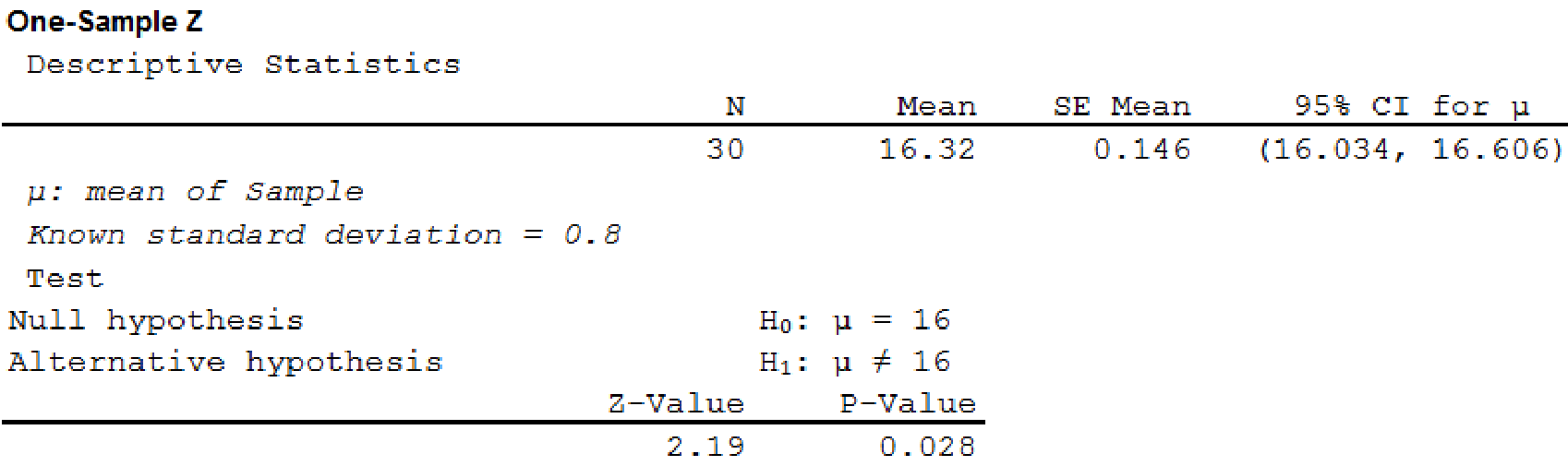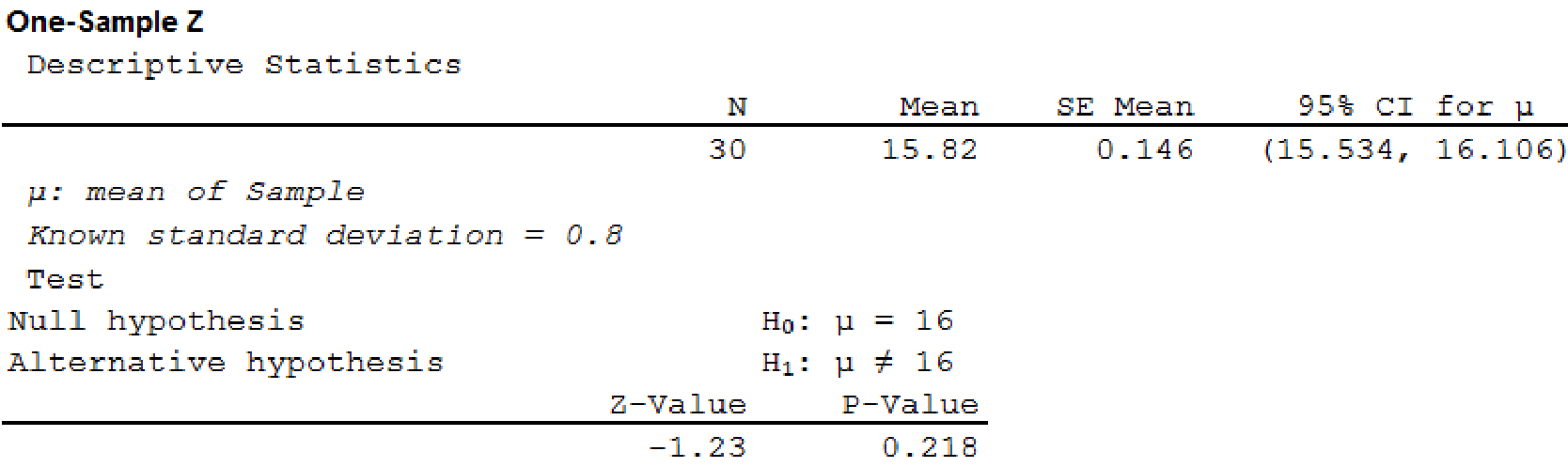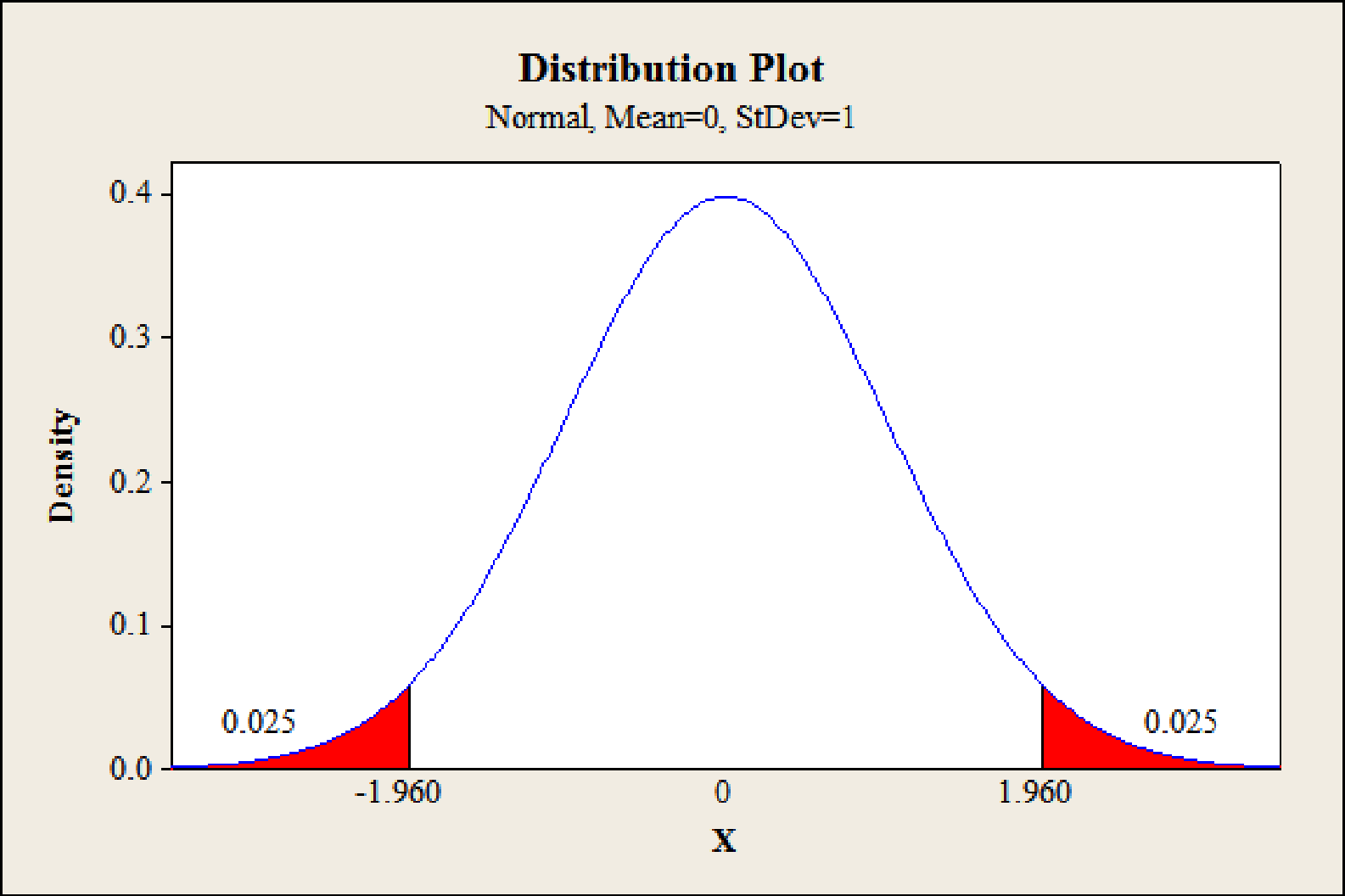# A production line operates with a mean filling weight of 16 ounces per container. Overfilling or underfilling presents a serious problem and when detected requires the operator to shut down the production line to readjust the filling mechanism. From past data, a population standard deviation σ = .8 ounces is assumed. A quality control inspector selects a sample of 30 items every hour and at that time makes the decision of whether to shut down the line for readjustment. The level of significance is α = .05. a. State the hypothesis test for this quality control application. b. If a sample mean of x ¯ = 16.32 ounces were found, what is the p -value? What action would you recommend? c. If a sample mean of x ¯ = 15.82 ounces were found, what is the p -value? What action would you recommend? d. Use the critical value approach. What is the rejection rule for the preceding hypothesis testing procedure? Repeat parts (b) and (c). Do you reach the same conclusion?13th Edition
Anderson
Publisher: CENGAGE L
ISBN: 978130588188413th Edition
Anderson
Publisher: CENGAGE L
ISBN: 9781305881884

#### Solutions

Chapter 9, Problem 60SE
Textbook Problem

## A production line operates with a mean filling weight of 16 ounces per container. Overfilling or underfilling presents a serious problem and when detected requires the operator to shut down the production line to readjust the filling mechanism. From past data, a population standard deviation σ = .8 ounces is assumed. A quality control inspector selects a sample of 30 items every hour and at that time makes the decision of whether to shut down the line for readjustment. The level of significance is α = .05. a. State the hypothesis test for this quality control application. b. If a sample mean of x ¯ = 16.32 ounces were found, what is the p-value? What action would you recommend? c. If a sample mean of x ¯ = 15.82 ounces were found, what is the p-value? What action would you recommend? d. Use the critical value approach. What is the rejection rule for the preceding hypothesis testing procedure? Repeat parts (b) and (c). Do you reach the same conclusion?

Expert Solution

a.

To determine

Identify the null and alternative hypotheses for the given situation.

The null and alternative hypotheses are given below:

Null hypothesis: H0:μ=16

Alternative hypothesis: Ha:μ16 (claim)

### Explanation of Solution

The given information is that the mean filling weight is 16 ounces.

Here, the claim is that overfilling or underfilling exists. This can be written as μ16. The complement of the claim is μ=16. In the given experiment, the alternative hypothesis indicates the claim.

The null and alternative hypotheses are given below:

Null hypothesis:

H0:μ=16

Alternative hypothesis:

Ha:μ16 (claim)

Expert Solution

b.

To determine

Find the p-value when x¯=16.32 and explain the recommended action.

The p-value is 0.028.

The conclusion is that there is evidence that the overfilling or underfilling exists.

### Explanation of Solution

Calculation:

The given information is that n=30, x¯=16.32, and σ=0.8.

Test statistic and p-value:

Software Procedure:

A step-by-step procedure to obtain the test statistic using MINITAB software:

• Choose Stat > Basic Statistics > 1-Sample Z.
• In Summarized data, enter the sample size as 30 and mean as 16.32.
• In Standard deviation, enter a value for s as 0.8.
• In Perform hypothesis test, enter the test mean as 16.
• Check Options; enter Confidence level as 95%.
• Choose not equal in alternative.
• Click OK.

Output using MINITAB software is given below:From the output, the test statistic is 2.19, and the p-value is 0.028.

Rejection rule:

If p-valueα, reject the null hypothesis.

If p-value>α, do not reject the null hypothesis.

Conclusion:

Here, the p-value is less than the level of significance.

That is, p-value(=0.028)<α(=0.05).

By the rejection rule, the null hypothesis is rejected.

Hence, there is evidence that the overfilling or underfilling exists. That is, the production line operates properly. Thus, the actions shut down and readjust the production line would be recommended.

Expert Solution

c.

To determine

Find the p-value when x¯=15.82 and explain the recommended action.

The p-value is 0.218.

The conclusion is that there is no evidence that the overfilling or underfilling exists.

### Explanation of Solution

Calculation:

The given information is that n=30, x¯=15.82, and σ=0.8.

Test statistic and p-value:

Software Procedure:

A step-by-step procedure to obtain the test statistic using MINITAB software:

• Choose Stat > Basic Statistics > 1-Sample Z.
• In Summarized data, enter the sample size as 30 and mean as 15.82.
• In Standard deviation, enter a value for s as 0.8.
• In Perform hypothesis test, enter the test mean as 16.
• Check Options; enter Confidence level as 95%.
• Choose not equal in alternative.
• Click OK.

Output using MINITAB software is given below:From the output, the test statistic is –1.23, and the p-value is 0.218.

Conclusion:

Here, the p-value is greater than the level of significance.

That is, p-value(=0.218)>α(=0.05).

By the rejection rule, the null hypothesis is not rejected.

Hence, there is no evidence that the overfilling or underfilling exists. That is, the production line does not operate properly. Thus, the action to continue the production process would be recommended.

Expert Solution

d.

To determine

Find the rejection rule using the critical value approach.

Find the conclusion when x¯=16.32 and x¯=15.82 and then compare the result with parts (b) and (c).

The rejection rule using the critical value is as follows:

If z1.96 or z1.96, reject the null hypothesis.

The conclusion for x¯=16.32; there is evidence that the overfilling or underfilling exists.

The conclusion for x¯=15.82; there is no evidence that the overfilling or underfilling exists.

### Explanation of Solution

Calculation:

The rejection rule for a two-tailed test using the critical value is as follows:

If zzα or zzα, reject the null hypothesis.

Critical value:

Software Procedure:

A step-by-step procedure to obtain the critical value using MINITAB software:

• Choose Graph > Probability Distribution Plot choose View Probability> OK.
• From Distribution, choose ‘Normal’ distribution.
• Click the Shaded Area tab.
• Choose Probability Value and Both Tails for the region of the curve to shade.
• Enter the Probability value as 0.05.
• Click OK.

Output using MINITAB software is given below:From the output, the critical value is ±1.96.

Rejection rule:

If z1.96 or z1.96, reject the null hypothesis.

Conclusion for x¯=16.32:

Here, the test statistic is greater than the critical value.

That is, z(=2.19)>zα(=1.96).

By the rejection rule, the null hypothesis is rejected.

Hence, there is evidence that the overfilling or underfilling exists. That is, the production line operates properly. Thus, the actions shut down and readjust the production line would be recommended.

Conclusion for x¯=15.82:

Here, the test statistic is greater than the critical value.

That is, z(=1.23)>zα(=1.96).

By the rejection rule, the null hypothesis is not rejected.

Hence, there is no evidence that the overfilling or underfilling exists. That is, the production line does not operate properly. Thus, the action to continue the production process would be recommended.

### Want to see more full solutions like this?

Subscribe now to access step-by-step solutions to millions of textbook problems written by subject matter experts!

### Want to see more full solutions like this?

Subscribe now to access step-by-step solutions to millions of textbook problems written by subject matter experts!

Find more solutions based on key concepts
TIME FOR A LUMP SUM TO DOUBLE If you deposit money today in an account that pays 6.5% annual interest how long ...

Fundamentals of Financial Management, Concise Edition (with Thomson ONE - Business School Edition, 1 term (6 months) Printed Access Card) (MindTap Course List)

Sum of Square (SS), variance and standard deviation

Statistics for The Behavioral Sciences (MindTap Course List)

Using the power series for cos x, the sum of the series is: cos(0.0625) cos(0.25) cos(0.5)

Study Guide for Stewart's Single Variable Calculus: Early Transcendentals, 8th

The radius of convergence of is: 1 3 ∞

Study Guide for Stewart's Multivariable Calculus, 8th

Is it true that f(g+h)=fg+fh?

Single Variable Calculus: Early Transcendentals

In Exercises 4562, find the values of x that satisfy the inequality (inequalities). 45. 2x + 4 8

Applied Calculus for the Managerial, Life, and Social Sciences: A Brief Approach

Round all answers to two decimal places. Exponential Regression In Exercises S-7 through S-14, use exponential ...

Functions and Change: A Modeling Approach to College Algebra (MindTap Course List)

Practice Graph each inequality. 4x-3y6

College Algebra (MindTap Course List)

Construct a stem-and-leaf display for the following data.

Modern Business Statistics with Microsoft Office Excel (with XLSTAT Education Edition Printed Access Card) (MindTap Course List)

In Problems 120 find either F(s) or f(t), as indicated. 9. {(1et+3e4t)cos5t}

A First Course in Differential Equations with Modeling Applications (MindTap Course List)